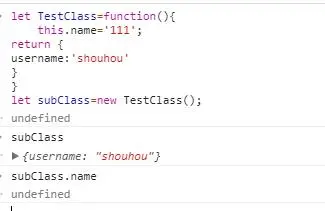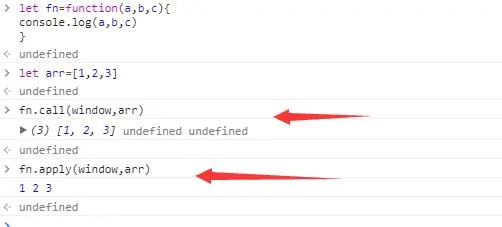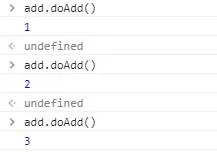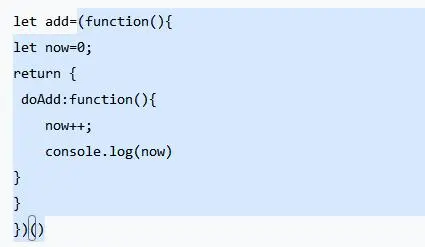# JavaScript：面试频繁出现的几个易错点

18,864

## 2.面向对象编程

``````//面向对象
//定义人（姓名）
let People=function(name){
this.name=name;
}
//动作
People.prototype={
eat:function(someThing){
console.log(`\${this.name}吃\${someThing}`);
}
}
//守候是个人，所以要创建一个人(new一次People)
let shouhou=new People('守候','男',24);
shouhou.eat('火锅');

//面向过程
let eat=function(who,someThing){
console.log(`\${who}吃\${someThing}`);
}
eat('守候','火锅');``````

``````//面向对象
shouhou.coding=function(){
console.log(this.name+'写代码');
}
shouhou.coding();
//面向过程
let coding=function(who){
console.log(who+'写代码');
}
coding('守候');``````

## 3.this

### 3-1.普通函数调用

``````let username='守候'
function fn(){
}
fn();``````

``````var username='守候'
function fn(){
}
fu();
//---------------
function fn(){
}
fn();
//可以理解为
//window.fn();``````

### 3-2.对象函数调用

``````window.b=2222
let obj={
a:111,
fn:function(){
}
}
obj.fn();``````

``````let obj1={
a:222
};
let obj2={
a:111,
fn:function(){
}
}
obj1.fn=obj2.fn;
obj1.fn();//222``````

### 3-3.构造函数调用

``````let TestClass=function(){
this.name='111';
}
let subClass=new TestClass();
subClass.name='守候';
console.log(subClass.name);//守候
let subClass1=new TestClass();
console.log(subClass1.name)//111``````### 3-4.apply和call调用

apply和call简单来说就是会改变传入函数的this。

``````let obj1={
a:222
};
let obj2={
a:111,
fn:function(){
}
}
obj2.fn.call(obj1);``````

### 3-5.箭头函数调用

``````let obj={
a:222,
fn:function(){
setTimeout(function(){console.log(this.a)})
}
};
obj.fn();//undefined``````

``````let obj={
a:222,
fn:function(){
setTimeout(()=>{console.log(this.a)});
}
};
obj.fn();//222``````

## 4.call和apply

`call``apply` 的作用，完全一样，唯一的区别就是在参数上面。
`call` 接收的参数不固定，第一个参数是函数体内 `this` 的指向，第二个参数以下是依次传入的参数。
apply接收两个参数，第一个参数也是函数体内 `this` 的指向。第二个参数是一个集合对象（数组或者类数组）

``````let fn=function(a,b,c){
console.log(a,b,c);
}
let arr=[1,2,3];````````````let obj1={
a:222
};
let obj2={
a:111,
fn:function(){
}
}
obj2.fn.call(obj1);``````

`call``apply` 两个主要用途就是

1.改变 `this` 的指向（把 `this``obj2` 指向到 `obj1`

2.方法借用（ `obj1` 没有 `fn` ，只是借用 `obj2` 方法）

## 5.闭包

``````let add=(function(){
let now=0;
return {
now++;
console.log(now);
}
}
})()``````## 6.小结

-------------------------华丽的分割线--------------------